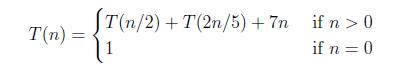# GATE | GATE CS 2021 | Set 1 | Question 40

• Difficulty Level : Medium
• Last Updated : 24 May, 2021

Consider the following recurrence relation.Which one of the following options is correct?
(A) T(n) = Θ(n5/2)
(B) T(n) = Θ(nlogn)
(C) T(n) = Θ(n)
(D) T(n) = Θ((logn)5/2)

Explanation: Given, recurrence relation can be written as,
T(n) = T(n/2) + T(2n/5) + 7n
T(n) = T((5/2)n/5) + T(2n/5) + 7n

Since, sum of numerator (5/2+2 = 4.5) is less than denominator (5), so time complexity would be function itself.

Hence, T(n) = Θ(7n) = Θ(n)

Quiz of this Question

My Personal Notes arrow_drop_up
Recommended Articles
Page :